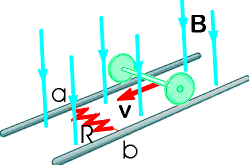Ask question

# Find the value of r so the line that passes through each pair of points has the given slope. (r, -5), (3, 13), m = 8# Find the value of r so the line that passes through each pair of points has the given slope. (r, -5), (3, 13), m = 8

Question
Linear equations and graphsasked 2021-01-15
Find the value of r so the line that passes through each pair of points has the given slope. (r, -5), (3, 13), m = 8

## Answers (1)2021-01-16
1:
$$m=(y^2-y^1)/(x^2-x^1)$$
2:
$$8=(13-(-5))/(3-r)$$
3:
$$8=18/(3-r)$$
8(3-r)=18
24-8r=18
8r=6
$$r=6/4$$

### Relevant Questionsasked 2021-05-16
Consider the curves in the first quadrant that have equationsy=Aexp(7x), where A is a positive constant. Different valuesof A give different curves. The curves form a family,F. Let P=(6,6). Let C be the number of the family Fthat goes through P.
A. Let y=f(x) be the equation of C. Find f(x).
B. Find the slope at P of the tangent to C.
C. A curve D is a perpendicular to C at P. What is the slope of thetangent to D at the point P?
D. Give a formula g(y) for the slope at (x,y) of the member of Fthat goes through (x,y). The formula should not involve A orx.
E. A curve which at each of its points is perpendicular to themember of the family F that goes through that point is called anorthogonal trajectory of F. Each orthogonal trajectory to Fsatisfies the differential equation dy/dx = -1/g(y), where g(y) isthe answer to part D.
Find a function of h(y) such that x=h(y) is the equation of theorthogonal trajectory to F that passes through the point P.asked 2021-05-14
For the equation (-1,2), $$y= \frac{1}{2}x - 3$$, write an equation in slope intercept form for the line that passes through the given point and is parallel to the graph of the given equation.asked 2020-11-20
A line passes through the point (2, 1) and has a slope of $$\frac{-3}{5}$$.
What is an equation of the line?
A.$$y-1=\frac{-3}{5}(x-2)$$
B.$$y-1=\frac{-5}{3}(x-2)$$
C.$$y-2=\frac{-3}{5}(x-1)$$
D.$$y-2=\frac{-5}{3}(x-1)$$asked 2020-12-13
Find the value of x or y so that the line passing through the given points has the given slope. (-9,y),(0,-3),$$m=\frac{-7}{9}$$asked 2021-03-07
Find the value of x or y so that the line passing through the given points has the given slope. (9, 3), (-6, 7y), $$m = 3$$asked 2021-03-21
In the figure below, the rolling axle, 1.43 m long, is pushed along horizontal rails at a constant speed v = 3.36 m/s.A resistor R = 0.325 ohm is connected to the rails at points a and b, directly opposite each other. (The wheels make good electrical contact with the rails, and so the axle, rails, and R form a closed-loop circuit. The only significant resistance in the circuit is R.) There is a uniform magnetic field B = 0.0850 T vertically downward. Calculate the induced current I in the resistor and what horizontal force F is required to keep the axle rolling at constant speed?asked 2021-05-27
Use the given conditions to write an equation for the line in point-slope form and in slope-intercept form.
Passing through (-2,-8) and parallel to the line whose equation is y=-3x+4
Write an equation for the line in point-slope form and slope-intercept form.asked 2021-02-25
We will now add support for register-memory ALU operations to the classic five-stage RISC pipeline. To offset this increase in complexity, all memory addressing will be restricted to register indirect (i.e., all addresses are simply a value held in a register; no offset or displacement may be added to the register value). For example, the register-memory instruction add x4, x5, (x1) means add the contents of register x5 to the contents of the memory location with address equal to the value in register x1 and put the sum in register x4. Register-register ALU operations are unchanged. The following items apply to the integer RISC pipeline:
a. List a rearranged order of the five traditional stages of the RISC pipeline that will support register-memory operations implemented exclusively by register indirect addressing.
b. Describe what new forwarding paths are needed for the rearranged pipeline by stating the source, destination, and information transferred on each needed new path.
c. For the reordered stages of the RISC pipeline, what new data hazards are created by this addressing mode? Give an instruction sequence illustrating each new hazard.
d. List all of the ways that the RISC pipeline with register-memory ALU operations can have a different instruction count for a given program than the original RISC pipeline. Give a pair of specific instruction sequences, one for the original pipeline and one for the rearranged pipeline, to illustrate each way.
Hint for (d): Give a pair of instruction sequences where the RISC pipeline has “more” instructions than the reg-mem architecture. Also give a pair of instruction sequences where the RISC pipeline has “fewer” instructions than the reg-mem architecture.asked 2020-12-21
Given linear equation y = -8-4x
a. find the y-intercept and slope.
b. determine whether the line slopes upward, slopes downward, or is horizontal, without graphing the equation.
c. use two points to graph the equation.asked 2021-01-16
Write an equation of the line that passes through the points.
(2, 5), (0, 5)
...